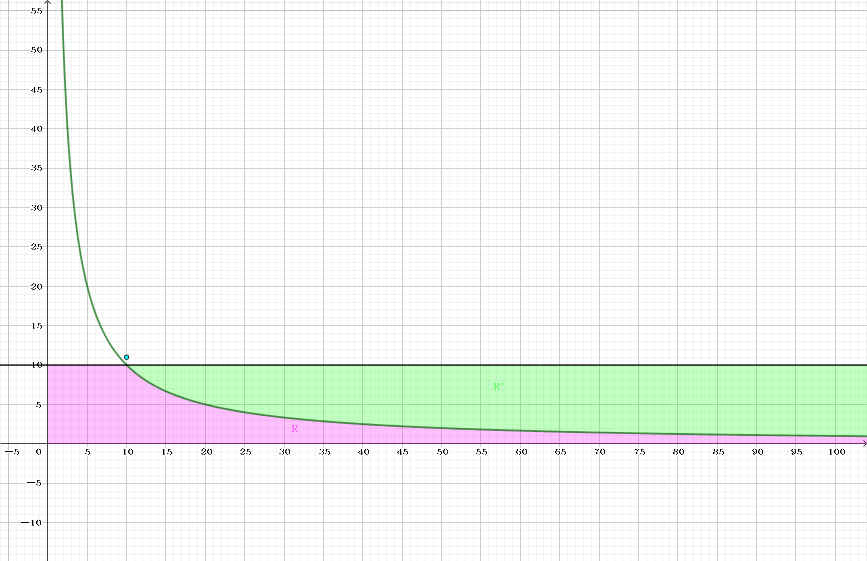# x义x的博客

……就算是单程票，也是可以的吧？

0%• 取出栈顶向量弹出，不断加上它，直到还差一步就会走进 $R$。

• 每走一步都可能带来一些贡献。如图，贡献是 $xv+(v+1)(u-1)/2$。（皮克定理）• 不断弹出栈顶，直到加上栈顶刚好走不进 $R$，称它为 $r$。设最近一次弹的是 $l$（它也有可能是上一步的栈顶向量）。现在的情况是，加上 $l$ 刚好走进 $R$，加上 $r$ 刚好走不进 $R$。• 开始二分，用 Stern-Brocot Tree 搞出一个 $l,r$ 的中间向量，也就是 $(l_x+r_x,l_y+r_y)$ 称为 $mid$。

• 如果 $mid$ 走一步不会进 $R$，那么 $mid$ 进栈并把 $r\leftarrow mid$ 继续二分。
• 否则，
• 如果 $r\le f’(x+x_{mid})$，那么如果继续二分就会找不到任何一步不进 $R$ 的向量，直接停止二分。• ↑ 可以发现如果 $r\le f’(x+x_{mid})$，$mid,r$ 的中间向量 $midmid$ 显然一步进 $R$，而且因为 $f’$ 单调增，$r\le f’(x+x_{midmid})$ 也一定满足……进入了死结。
• 否则 $l\leftarrow mid$ 继续二分。

• $(x,y)$ 从 $(\lfloor\dfrac{n}{B}\rfloor,B+1)$ 开始。可以发现所用斜率必然是 $[-1,0]$ 之间，于是栈里初始化为 $(1,0),(1,1)$。

• 为了方便可以改为存向量的绝对值。（真的方便吗？）